# Branching processes, regularity of

(diff) ← Older revision | Latest revision (diff) | Newer revision → (diff)

A property of branching processes ensuring that the number of particles at any moment of time is finite. The problem of the regularity of a branching process is usually reduced to the problem of uniqueness of the solution of some differential or integral equation. For instance, in a continuous-time branching process the differential equation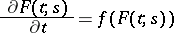with the initial condition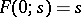has a unique solution if and only if, for any, the integral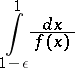is divergent. In the branching Bellman–Harris process the generating functionof the number of particles is the solution of the non-linear integral equation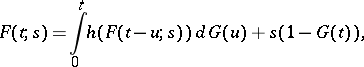(*)

where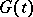is the distribution function of the lifetimes of particles andis the generating function of the number of daughter particles ( "direct descendants" ) of a single particle. If, for given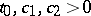and an integer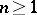, the inequalitiesare valid for all, the solution of equation (*) is unique if and only if the equationwith initial conditions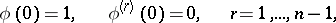has a unique solutionFor a branching process described by equation (*) to be regular, it is necessary and sufficient for the integralto diverge for any.

How to Cite This Entry:
Branching processes, regularity of. Encyclopedia of Mathematics. URL: http://encyclopediaofmath.org/index.php?title=Branching_processes,_regularity_of&oldid=11240
This article was adapted from an original article by B.A. Sevast'yanov (originator), which appeared in Encyclopedia of Mathematics - ISBN 1402006098. See original article